# M8-S10: Modelling Radioactive Decay (Calculation)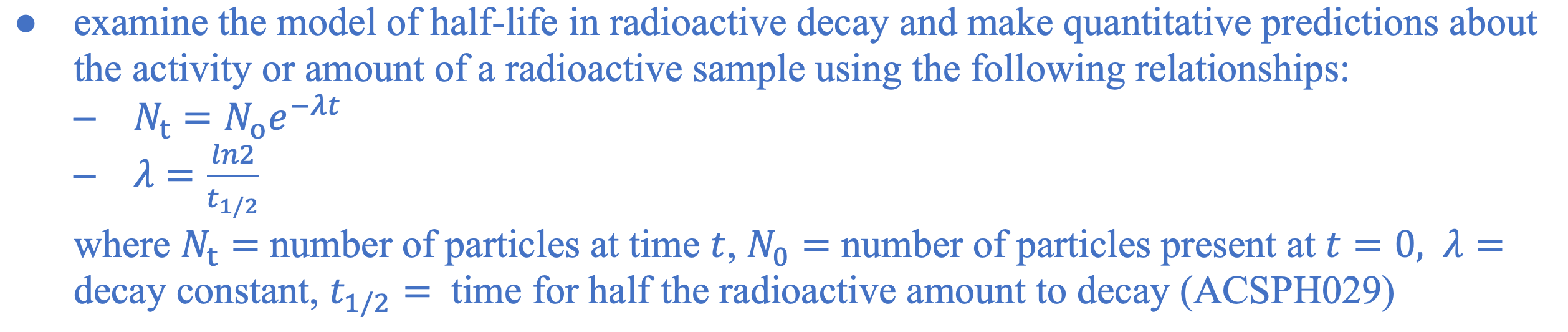### Using Mathematics to Model Radioactive Decay

• Radioactive decay cannot be accurately modelled using a mathematical equation as it occurs on a small scale and is dependent on the activity of every atom or nucleus. Thus, what it is taught in HSC Physics is merely a glimpse of what quantitative nuclear physics looks like.
• Radioactive decay can be modelled exponentially by the equation: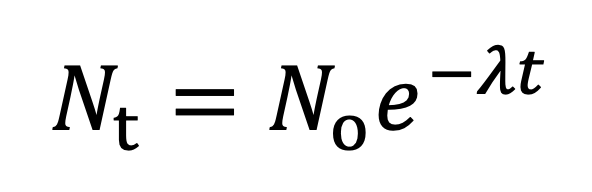where Nt is the number of nuclides present after time t. No is the initial number of nuclides when t = 0. l is the decay constant which is specific for a particular isotope.• The decay constant provides an easy to way to compare the half-life of different decay processes.
• A large decay constant corresponds to a short half-life – more nuclides are disintegrated per second.
• A small decay constant corresponds to a long half-life – fewer nuclides are disintegrated per second.

### Half-life

• Half-life of a radioisotope is the time taken for half the amount of nuclide to decay to form daughter isotopes. This occurs when:Where t1/2 is the half-life which stays constant throughout a nuclide’s decay process. However, the amount of nuclide disintegrated during each interval of half-life decreases throughout the process (see below).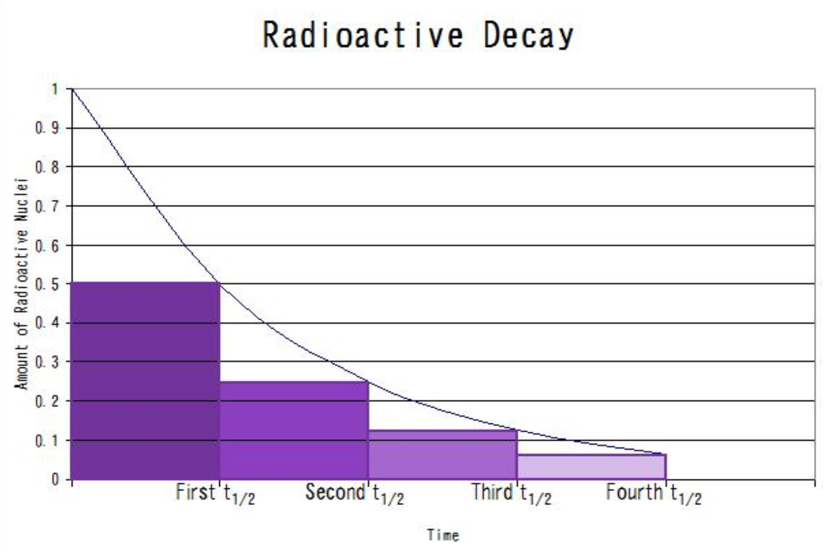Figure shows the change in radioactive nuclide over time as modelled by exponential decay. For every half-life interval the amount of parent nuclide is halved - hence the term 'half-life'.

• For example, a particular radioisotope will take t1/2 for 50% of its original quantity (No) to disintegrate. It will take 2t1/2 for 25%, 3t1/2 for 12.5% and so on.

### Activity/Disintegration Rate

• Activity of decay or a radioisotope can be characterised by the differential equation:Where A is activity of decay or rate of disintegration. Its SI unit is becquerel (Bq) and a commonly used non-SI unit is Curie (C). Bq is equivalent to per second (s-1) while C is equivalent to 3.7 x 1010 s-1.

Thus, the activity of radioactive decay changes with number of nuclides present. As the decay progresses, the activity decreases.

• As parent nuclides decay, the quantity of daughter nuclides increases. The increase in daughter nuclides is modelled logarithmically.• Sometimes the daughter nuclides can also be radioactive and thus can undergo radioactive decay simultaneously.Graph shows the decay process of iodine-135 to form xenon-135. Xe-135 is radioactive and forms stable caesium-135 through decay. Notice that the mass of Xe-135 increases initially but then follows exponential decay subsequently.

What units are suitable for radioactive decay calculation?

• Normally, SI units are always encouraged for performing calculations in physics. However, there is a degree of flexibility when calculating radioactive decay.
• Half-life or time can be provided in various units such as hours and years. They do not need to be converted into seconds. The unit you use depends on the decay constant and also the disintegration rate or activity.
• For example, if the decay constant has a unit of yr-1 then time values in the unit of year can be used in the same equation.
• Activity or disintegration rate can be provided in various units such as Bq (s-1), C, min-1 and hr-1. They do not need to be always converted to the SI unit of Bq. The unit you use depends on the decay constant and also the half-life you choose to use in the equation.
• For example, if the decay constant has a unit of yr-1 then the activity must be converted to yr-1

Example Questions

1. Technetium-99m has a decay constant of 3.2 x 10-5 s-1. What is its half-life in hours?

Solution:

Using the decay constant equation:2. An ancient wooden boat is discovered. The wood has a carbon-14 activity of 0.0058 Bq. A modern piece of wood has an activity of 0.30 Bq. The decay constant of carbon-14 is 1.24 x 10-4 yr-1.

How old is the wooden boat?

Solution:

The activity of radioactive decay is modelled by the equation:Assuming the modern piece of wood has No number of nuclides that are yet to decay: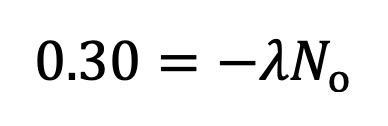For the old piece of wood: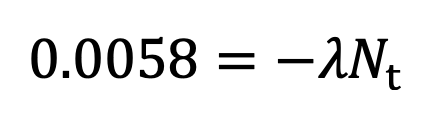By dividing the second by the first equation: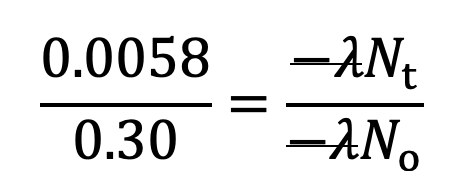Since: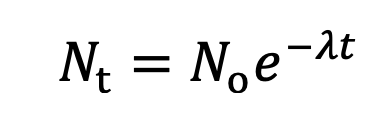Rearranging gives:Notice how the decay constant provided in the question has the unit 'per year'. As a result, the final answer (time) has the unit of years rather than the typical SI unit of seconds.

Practice Question 1

Determine the decay constant for carbon-14 if it has a half-life of 5730 years. (1 mark)

Practice Question 2

If Radium-223 has a half-life of 10.33 days, what time duration would it require for the activity associated with this sample to decrease 1.5% of its present value? (2 marks)

Practice Question 3

The disintegration rate of a sample of Co-60 is 6800 hr-1. If the half-life of Co-60 is 5.3 years, determine the quantity of atoms in this sample. (2 marks)